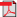# Search Results

1. E. Nyberg, J. Virtamo and S. Aalto, An Exact End-to-End Blocking Probability Algorithm for Multicast Networks, Performance Evaluation, vol. 54, no. 4, pp. 311-330, 2003(bib)
Abstract: We consider the calculation of blocking probabilities in multicast trees with dynamic membership. We extend the work by Karvo et al., where an approximate algorithm based on the Reduced Load Approximation was given to calculate end-to-end blocking for infinite sized user populations in multicast networks. The new end-to-end call blocking algorithm for an arbitrary sized user population is based on the known blocking probability algorithm in hierarchical multiservice access networks, where link occupancy distributions are alternately convolved and truncated. We show that the algorithm can be applied to multicast trees embedded in a network with an arbitrary topology carrying also non-multicast traffic. The resource sharing of multicast connections, however, requires the modification of the algorithm by using a new type of convolution, the OR-convolution. In addition, we discuss several different user population models for which the algorithm is applicable.